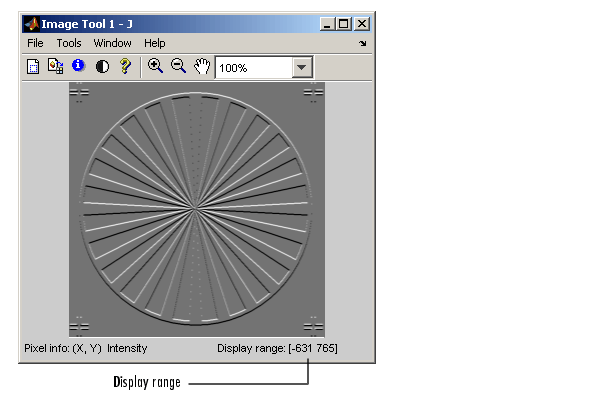Image Processing Toolbox User's GuideDisplaying Grayscale Images

To display a grayscale image, using either `imshow` or `imtool`, specify the image matrix as an argument. This documentation uses the variable name `I` to represent a grayscale image in the workspace.

• ```imshow(I)
```

or

• ```imtool(I)
```

Both functions display the image by scaling the intensity values to serve as indices into a grayscale colormap.

If `I` is `double`, a pixel value of 0.0 is displayed as black, a pixel value of 1.0 is displayed as white, and pixel values in between are displayed as shades of gray. If `I` is `uint8`, then a pixel value of 255 is displayed as white. If I is `uint16`, then a pixel value of 65535 is displayed as white.

Grayscale images are similar to indexed images in that each uses an m-by-3 RGB colormap, but you normally do not specify a colormap for a grayscale image. MATLAB displays grayscale images by using a grayscale system colormap (where R=G=B). By default, the number of levels of gray in the colormap is 256 on systems with 24-bit color, and 64 or 32 on other systems. (See Working with Different Screen Bit Depths for a detailed explanation.)

Displaying Grayscale Images That Have Unconventional Ranges

In some cases, the image data you want to display as a grayscale image might have a display range that is outside the conventional toolbox range (i.e., [0,1] for `single` or `double` arrays, [0,255] for `uint8` arrays, [0,65535] for `uint16` arrays, or [-32767,32768] for `int16` arrays). For example, if you filter a grayscale image, some of the output data might fall outside the range of the original data.

To display unconventional range data as an image, you can specify the display range directly, using this syntax for both the `imshow` and `imtool` functions.

• ```imshow(I,'DisplayRange',[low high])
```

or

• ```imtool(I,'DisplayRange',[low high])
```

If you use an empty matrix (`[]`) for the display range, these functions scale the data automatically, setting `low` and `high` to the minimum and maximum values in the array.

The next example filters a grayscale image, creating unconventional range data. The example calls `imtool` to display the image, using the automatic scaling option. If you execute this example, note the display range specified in the lower right corner of the Image Tool window.

• ```I = imread('testpat1.png');
J = filter2([1 2;-1 -2],I);
imtool(J,'DisplayRange',[]);```Displaying Indexed Images Displaying Binary Images